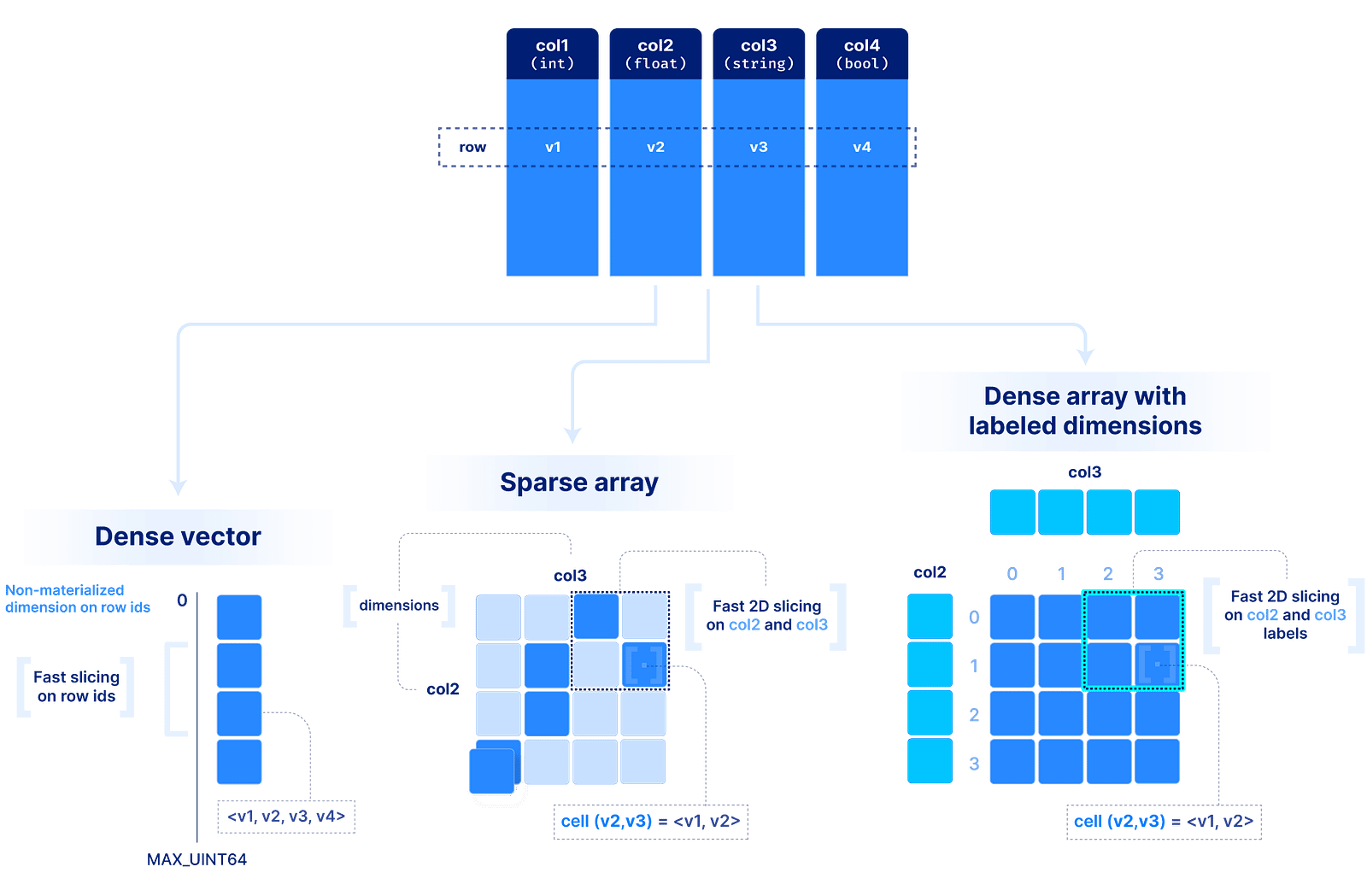# Use Cases

Multi-dimensional arrays have been around for a long time. However, there have been two misconceptions about arrays:
1. 1.
Arrays are used solely in scientific applications. This is mainly due to their massive use in Python, Matlab, R, machine learning and other scientific applications. There is absolutely nothing wrong with arrays capturing scientific use cases. On the contrary, such applications are important and challenging, and there is no relational database that can efficiently accommodate them.
2. 2.
Arrays are only dense. Most array systems (i.e., storage engines or databases) built before TileDB focused solely on dense arrays. Despite their suitability for a wide spectrum of use cases, dense arrays are inadequate for sparse problems, such as genomics, LiDAR and tables. Sparse arrays have been ignored and, therefore, no array system was able to claim universality.
The sky is the limit in terms of applicability for a system that supports both dense and sparse arrays. An image is a 2D dense array, where each cell is a pixel that can store the RGBA color values. Similarly a video is a 3D dense array, two dimensions for the frame images and a third one for the time. LiDAR is a 3D sparse array with float coordinates. Genomic variants can be modeled by a 3D array where the dimensions are the sample name (string), the chromosome (string) and the position (integer). Time series tick data can be modeled by a 2D array, with time and tick symbol as labeled dimensions (this can of course be extended arbitrarily to a ND dense or sparse array). Similarly, weather data can be modeled with a 2D dense array with float labels (the lat/lon real coordinates). Graphs can be modeled as (sparse 2D) adjacency matrices. Finally, a flat file can be stored as a simple 1D dense array where each cell stores a byte.Arrays can model a wide variety of applications
But what about tabular data? Arrays have a lot of flexibility here. In the most contrived scenario, we can store a table as a set of 1D arrays, one per column (similar to Parquet for those familiar with it). This is useful if we want to slice a range of rows at a time. Alternatively, we can store a table as a ND sparse array, using a subset of columns as the dimensions. That would allow rapid slicing on the dimension columns. Finally, we can use labeled dense arrays as explained above for the time series tick data.Arrays subsume tables

## What's next?

You may wonder how we can make all these decisions about dimensions vs. attributes and dense vs. sparse for each application. To answer that, we need to understand how dense and sparse arrays lay data out on the storage medium, and what factors affect performance when slicing, which is the focus of the key concepts and data format section:
In addition, check out the various TileDB use cases in more detail: